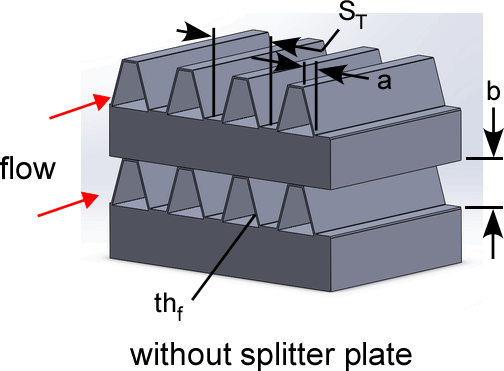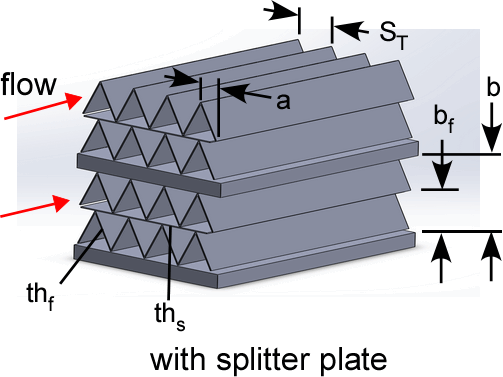Contents - Index

PF plain-fin geometryThe procedure:

CHX_geom_PF_plain_fin(TypeHX\$: S_T, a, th_f, th_s, b, b_f, D_h, theta, beta, A_fin\A)

Provides the geometric data associated with a plate finned - plain-fin type compact heat exchanger surface.  These data are from Kays and London (1994).

Inputs:

The only input is the string identifying the geometry TypeHX\$

3.01: 'pf_plain-fin_301'

3.97: 'pf_plain-fin_397'

9.03: 'pf_plain-fin_903'

11.11: 'pf_plain-fin_1111'

11.11a: 'pf_plain-fin_1111a'

14.77: 'pf_plain-fin_1477'

15.08: 'pf_plain-fin_1508'

19.86: 'pf_plain-fin_1986'

10.27T: 'pf_plain-fin_1027T'

11.94T: 'pf_plain-fin_1194T'

12.00T: 'pf_plain-fin_1200T'

16.96T: 'pf_plain-fin_1696T'

25.79T: 'pf_plain-fin_2579T'

30.33T: 'pf_plain-fin_3033T'

46.45T: 'pf_plain-fin_4645T'

Outputs:

S_T = transverse fin spacing (m or ft)

a = lateral shift in fin across gap (m or ft) (= 0 for straight fins, =S_T/2 for triangular fins)

th_f = thickness of fin material (m or ft)

th_s = thickness of splitter plate material (m or ft) (=0 for fins with no splitter)

b = plate gap (m or ft)

b_f = fin spacing (m or ft) (=b for fins with no splitter)

D_h = hydraulic diameter defined as 4 x minimum flow area x length/heat transfer area (m or ft)

theta = minimum flow area/frontal area for finned surface side only

beta = heat transfer area/volume (m^2/m^3 or ft^2/ft^3) for finned surface side only

A_fin\A = heat transfer area on fins/heat transfer area for finned surface side only

Example

\$UnitSystem SI Mass J K Pa

TypeHX\$= 'pf_plain-fin_4645T'

Call chx_geom_pf_plain_fin(TypeHX\$: S_T, a, th_f, th_s, b, b_f, D_h, theta, beta, A_fin\A)

{Solution is:

S_T = 0.0005467 [m]

a = 0.0002734 [m]

th_f = 0.000051 [m]

th_s = 0

b = 0.00254 [m]

b_f = 0.00254 [m]

D_h = 0.000805 [m]}

Related procedures include:

Nondimensional Functions

Coefficient of Heat Transfer

Pressure Drop

Compact Heat Exchangers